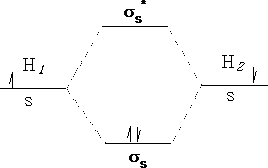# Ultraviolet Spectrocopy

What is spectroscopy? It is the interaction of light with atoms and molecules.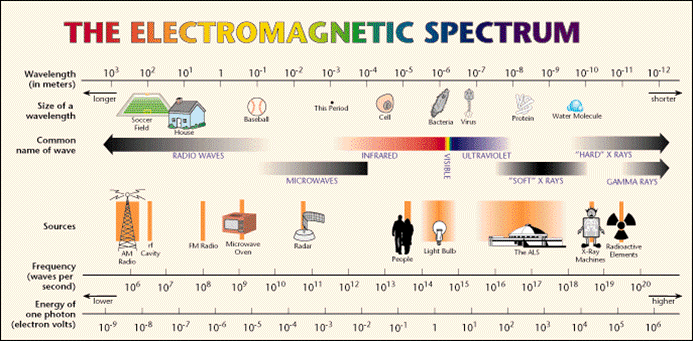If we look at the electromagnetic spectrum above we can see there is a huge range of different types of light, some we can see, but mostly ones we cannot. The visible spectrum is a very small part of the whole.

Light or electromagnetic radiation is best looked at in one of two ways i) waves ii) particles. Light behaves very much in this duality.

i) Look at the diagram of the wave :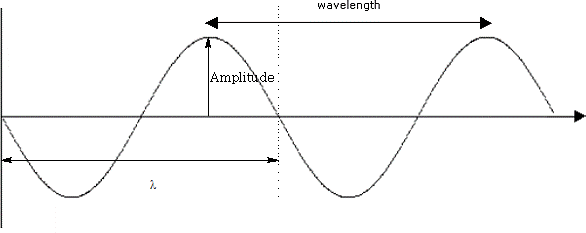λ = wavelength:

A = wave amplitude:

τ = period:

ν = frequency of oscillation:

c = velocity of light: (3.0 x 10 8 m/s)

ν = 1/ t

λν = c

ii) Light can be described also as a stream of energy packets or particles travelling with a high velocity. These particles are called photons . The frequency (?) can be related to the energy of the photons (E). Plank's Law gives us:

E = h ν

Where h is Plank's constant, a proportionality factor = 6.63 x 10-34 Js

Interaction of light with atoms

If continuous light is passed through a prism, the light is dispersed into its component wavelengths. If this light is then passed through cells containing the atoms under test, the emergent light is no longer continuous. Some of the light will have interacted with and have been absorbed by the atoms. The missing wavelengths can be detected by allowing the light emerging to fall on a detector which will record the image.

If atoms are heated to a sufficient high temperature, they emit light of certain wavelengths. These wavelengths can be used to identify the atoms; this spectrum is called an atomic emission spectrum.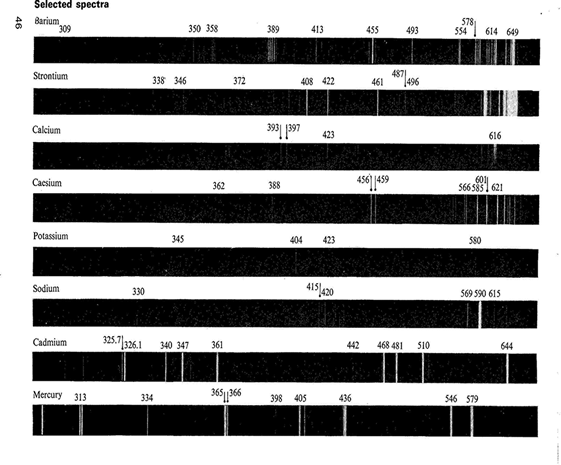How do these images appear? We are familiar with the concept of energy levels in atoms! Each energy level is quantized, i.e. energy can only have discrete values. The allowed energies are called atomic energy levels. A quantum of energy is absorbed when an atom is excited from a lower energy to a higher one.

Below is an energy diagram showing a quantum of energy being absorbed and transferred from a lower to a higher energy level. Different transitions will absorb different energies.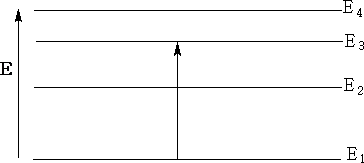In the energy diagram above the energy goes from E1 the lowest energy to E3 , transitional energy ΔE = E3 - E1.

If we now equate the energy transfers to the atoms, we need to look at the relative energies of the atomic orbitals.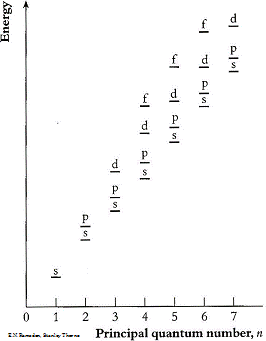Electrons can be promoted between levels if there is an empty space for them. E.g. Helium, electrons can be promoted to the p orbitals or above.e.g.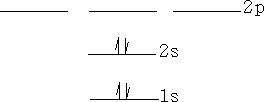Molecules have electronic energies also which are analogous to the energy levels in atoms. These molecular orbitals arise as we have already seen from the interactions of the atomic orbitals of the atoms forming the molecule. Consider a Hydrogen molecule; atom 1 interacts with atom 2 to produce two molecular orbitals in the molecule H2 .